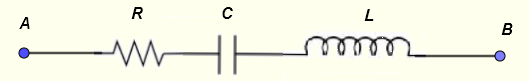# Series RLC circuit Impedance Calculator

  

A calculator to calculate the equivalent impedance of a resistor, a capacitor and and inductor in series. The calculator gives the impedance as a complex numbers in standard form , its modulus and argument which may be used to write the impedance in exponential and polar forms.

## Formulae for series RLC Circuit Used in the Calculator and their Units

We first give the formulas used in the series RLC calculator and the proof of these formulas is presented in the bottom part of the page.Let $f$ be the frequency, in Hertz, of the source voltage supplying the circuit.
and define the following parameters used in the calculations
$\omega = 2 \pi f$ , angular frequency in rad/s
$X_C = 1 / (\omega C)$ , the capacitor reactance in ohms $(\Omega)$
$X_L = \omega L$ , the inductor reactance in ohms $(\Omega)$
Let $Z$ be the equivalent impedance to the series RLC circuit shown above and write it in complex form as follows
$Z = r e^{j \theta}$ The formulae for the modulus $r$ and argument $\theta$ are given by (see proof at the bottom of the page)

Moduls: $|Z| = r = \sqrt {R^2 + (X_L - X_C)^2 }$ in ohms $(\Omega)$

Argument: $\theta = \arctan \left(\dfrac{X_L - X_C}{R} \right)$ in radians or degrees

## Use of the calculator

Enter the resistance, the capacitance, the inductance and the frequency as positive real numbers with the given units then press "calculate".

 Resistance R = 100 mΩ Ω KΩ MΩ Capacitance C = 10 pF nF μF mF F Inductance L = 10 μH mH H Frequency f = 1 GHz MHz kH Hz mHz

## Proofs of the Formulae for The series RLC Circuit

Let
$Z_R = R$ , $Z_C = \dfrac{1}{j \omega C}$ , $Z_L = j \omega L$
Apply the rule of impedances of a series ciruits to find the equivalent impedance $Z$ as follows
$Z = R + Z_C + Z_L$ Let
$X_L = \omega L$ and $X_C = \dfrac{1}{\omega C}$
and rewrite $Z$ as
$Z = R + \dfrac{1}{j \omega C} + j \omega L$
$Z = R + j ( - X_C + X_L )$
We now use the exponential form of complex number to write
$Z = r e^{j\theta}$
the modulus of $Z$ as
$r = \sqrt {R^2 + (X_L - X_C)^2 }$
the argument of $Z$ is given by
$\theta = \arctan \left(\dfrac{X_L - X_C}{R} \right)$

## Numerical Example Using the Above Formulas

Frequency $f = 1 \; kHz$ , $C = 10 \; \mu F$ , $L = 10 \; mH$ and $R = 100 \; \Omega$
$X_L = \omega L = 2 \pi f L = 2 \pi 10^3 10^{-2} = 62.83 \; \Omega$
$X_C = \dfrac{1}{\omega C} = \dfrac{1}{2 \pi f C} = \dfrac{1}{2 \pi 10^3 10^{-5} } = 15.92 \; \Omega$
Group imaginary terms
$Z = 100 + j ( 62.83 - 15.92 )$
Simplify
$Z = 100 + j ( 62.83 - 15.92 ) = 100 + 46.91 j$
Write the above in exponential form
$Z = \sqrt {100^2 + 46.91^2} e^{j \arctan{\dfrac{46.91}{100}}} = 110.45 \; e^{j 0.44}$
$Z$ written in phasor form
$Z = 110.45 \angle 0.44 \; rad = 110.45 \angle 25.13^{\circ}$

You may input the given values in the calculator and check the results.